# Ideal Gas Law

An ideal gas is defined as one in which all collisions between atoms or molecules are perfectly eleastic and in which there are no intermolecular attractive forces. One can visualize it as a collection of perfectly hard spheres which collide but which otherwise do not interact with each other. In such a gas, all the internal energy is in the form of kinetic energy and any change in internal energy is accompanied by a change in temperature.

An ideal gas can be characterized by three state variables: absolute pressure (P), volume (V), and absolute temperature (T). The relationship between them may be deduced from kinetic theory and is called the• n = number of moles
• R = universal gas constant = 8.3145 J/mol K
• N = number of molecules
• k = Boltzmann constant = 1.38066 x 10-23 J/K = 8.617385 x 10-5 eV/K
• k = R/NA
• NA = Avogadro's number = 6.0221 x 1023 /mol

The ideal gas law can be viewed as arising from the kinetic pressure of gas molecules colliding with the walls of a container in accordance with Newton's laws. But there is also a statistical element in the determination of the average kinetic energy of those molecules. The temperature is taken to be proportional to this average kinetic energy; this invokes the idea of kinetic temperature. One mole of an ideal gas at STP occupies 22.4 liters.

 Calculation
 Departure from an ideal gas: van der Waals equation of state
Index

Gas law concepts

Kinetic theory concepts

 HyperPhysics***** Thermodynamics R Nave
Go Back

# Molecular Constants

In the kinetic theory of gases, there are certain constants which constrain the ceaseless molecular activity. Gas properties are described in terms of state variables.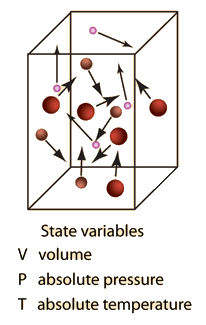A given volume V of any ideal gas will have the same number of molecules. The mass of the gas will then be proportional to the molecular mass. A convenient standard quantity is the mole, the mass of gas in grams equal to the molecular mass in amu. Avogadro's number is the number of molecules in a mole of any molecular substance. NA=Avogadro's number=6.0221 x 1023/mole The average translational kinetic energy of any kind of molecule in an ideal gas is given byIndex

Kinetic theory concepts

 HyperPhysics***** Thermodynamics R Nave
Go Back

# State Variables

A state variable is a precisely measurable physical property which characterizes the state of a system, independently of how the system was brought to that state. It must be inherently single-valued to characterize a state. For example in the heat-work example, the final state is characterized by a specific temperature (a state variable) regardless of whether it was brought to that state by heating, or by having work done on it, or both.

Common examples of state variables are the pressure P, volume V, and temperature T. In the ideal gas law, the state of n moles of gas is precisely determined by these three state variables. If a property, e.g., enthalpy H, is defined as a combination of other state variables, then it too is a state variable. Enthalpy is one of the four "thermodynamic potentials", and the other three, internal energy U, Helmholtz free energy F and Gibbs free energy G are also state variables. The entropy S is also a state variable.

Some texts just use the term "thermodynamic variable" instead of the description "state variable".

Index

Kinetic theory concepts

 HyperPhysics***** Thermodynamics R Nave
Go Back

# The Mole

A mole (abbreviated mol) of a pure substance is a mass of the material in grams that is numerically equal to the molecular mass in atomic mass units (amu). A mole of any material will contain Avogadro's number of molecules. For example, carbon has an atomic mass of exactly 12.0 atomic mass units -- a mole of carbon is therefore 12 grams. For an isotope of a pure element, the mass number A is approximately equal to the mass in amu. The accurate masses of pure elements with their normal isotopic concentrations can be obtained from the periodic table.

One mole of an ideal gas will occupy a volume of 22.4 liters at STP (Standard Temperature and Pressure, 0°C and one atmosphere pressure).# Standard Temperature and Pressure

STP is used widely as a standard reference point for expression of the properties and processes of ideal gases. The standard temperature is the freezing point of water and the standard pressure is one standard atmosphere. These can be quantified as follows:

Standard temperature: 0°C = 273.15 K

Standard pressure = 1 atmosphere = 760 mmHg = 101.3 kPa

Standard volume of 1 mole of an ideal gas at STP: 22.4 liters

Index

Kinetic theory concepts

 HyperPhysics***** Thermodynamics R Nave
Go Back

# Gauge Pressure

Does the flat tire on your automobile have zero air pressure? If it is completely flat, it still has the atmospheric pressure air in it. To be sure, it has zero useful pressure in it, and your tire gauge would read zero pounds per square inch. Most gauges read the excess of pressure over atmospheric pressure and this excess is called "gauge pressure". While a useful measurement for many practical purposes, it must be converted to absolute pressure for applications like the ideal gas law.

Since a partial vacuum will be below atmospheric pressure, the phrase "negative pressure" is often used. Certainly there is no such thing as a negative absolute pressure, but small decreases in pressure are commonly used to entrain fluids in sprayers, in carburetors for automobiles, and many other applications. In the case of respiration, we say that the lungs produce a negative pressure of about -4 mmHg to take in air, which of course means a 4 mmHg decrease from the surrounding atmospheric pressure.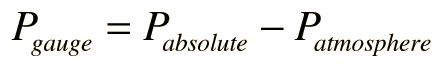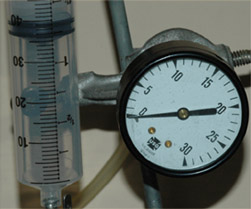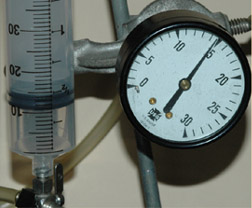When a system is at atmospheric pressure like the left image above, the gauge pressure is said to be zero. In this image, the system has been opened so that it is at equilibrium with the atmosphere. In the right image, the system has been closed and the plunger pushed down until the pressure reads about 15 lb/in2. This implies that the absolute pressure has been approximately doubled by compressing the gas to half its volume (ideal gas law). Standard atmospheric pressure in these U.S. common units is 14.7 lb/in2, so this must be added to the gauge pressure above to get the absolute pressure.

Index

Kinetic theory concepts

 HyperPhysics***** Thermodynamics R Nave
Go Back

# Ideal Gas Law with Constraints

For the purpose of calculations, it is convenient to place the ideal gas law in the form: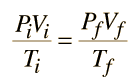where the subscripts i and f refer to the initial and final states of some process. If the temperature is constrained to be constant, this becomes: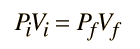which is referred to as Boyle's Law.

If the pressure is constant, then the ideal gas law takes the form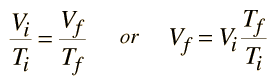which has been historically called Charles' Law. It is appropriate for experiments performed in the presence of a constant atmospheric pressure.

All the possible states of an ideal gas can be represented by a PvT surface as illustrated below. The behavior when any one of the three state variables is held constant is also shown.Index

Gas law concepts

Kinetic theory concepts

 HyperPhysics***** Thermodynamics R Nave
Go Back